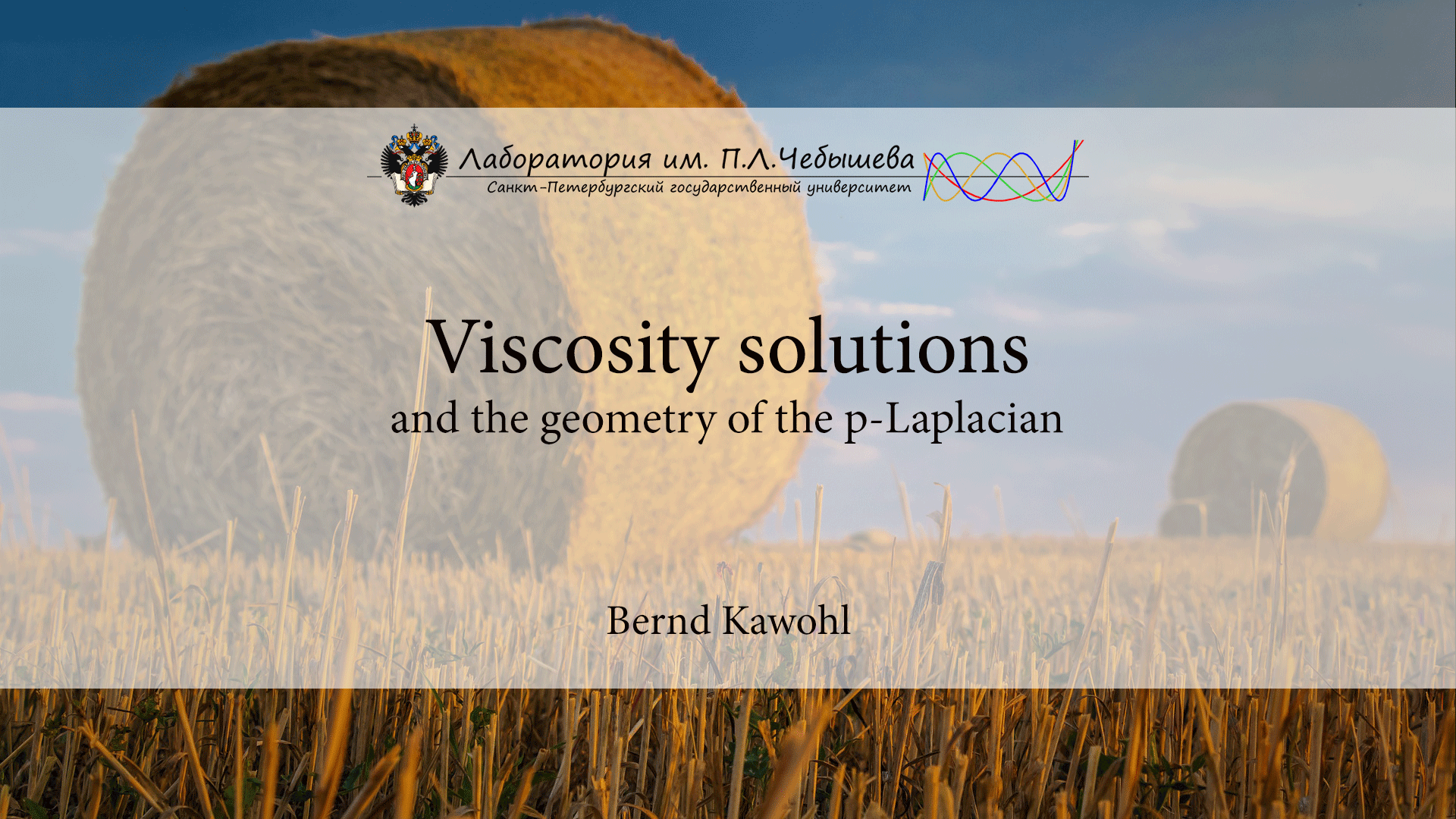# Viscosity solutions and the geometry of the p-Laplacian

Курс
Предмет:
Лектор:Viscosity solutions have become an important tool in the study of degenerate elliptic equations such as |∇u| 2 = 1 or −∆−pu :=div(|∇u| p−2∇u) = 1. Both equations can be regularized by adding −ε∆u to the left hand side. Then the equations become more elliptic and one can study the limit of their solutions as ε → 0. I believe that this idea goes back to Kruzkov. The p-Laplacian operator is usually defined for p ∈ (1,∞) and becomes more degenerate when p = 1 and p = ∞. Partial differential equations involving the 1-Laplacian or ∞-Laplacian lead to interesting geometric questions. The first eigenvalue of the ∞-Laplacian, for instance, is related to the inradius of the underlyimng domain. In my lectures I will study equations like −∆pu = 1, −∆N p u := 1 p |∇u| 2 − p∆pu = 1 or −∆pu = λ|u| p−2u and their limits as p → 1 or p → ∞. As byproducts we shall study a partitioning problem and Cheeger sets and learn about different approaches to mathematical image processing. I will also address overdetermined boundary value problems for degenerate elliptic equations and show how they relate to Pohozaev identities. Many definitions in the theory of viscosity solutions become clearer when they are tested on ordinary differential equations. In this context the theory is closely related to singular perturbation theory with its boundaryand transition-layers.

Аннотация миникурса

2